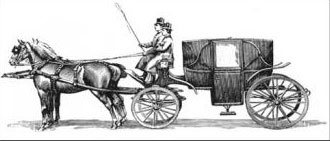Science, Maths & Technology

### Become an OU studentStart this free course now. Just create an account and sign in. Enrol and complete the course for a free statement of participation or digital badge if available.

# 3 Work on your own mathematics

Two activities are given below. You are asked to work on them in turn and to record not only your working, but observations on what you notice about your emotions as you work through step by step.

## Activity 3 Constrained numbers

Starter

Write down a number between 2 and 4. And another. And another.

### Discussion

Experience suggests that there is a significant difference between being asked for three examples all at once, and being asked for three one after the other with pauses for the construction at each stage. By the third request, many learners are ‘feeling bored’ and so challenge themselves by constructing more complex examples. The point of the task is to get learners to become aware of the range of choices open to them and, more specifically, the general class of possibilities from which they can choose, so they do not jump at the first thing that comes to mind

Write down a decimal number that lies between 2 and 3.

Now add a further constraint. Write down a decimal number that lies between 2 and 3, and does not use the digit 5.

Now add a further constraint. Write down a decimal number that lies between 2 and 3, and does not use the digit 5 but does use the digit 8.

Add a further constraint. Write down a decimal number that lies between 2 and 3, and does not use the digit 5 but does use the digit 8, and is as close to 2½ as possible.

Variant 1

Write down a fraction between 4 and 5.

Write down a fraction between 4 and 5 that uses each digit 0 to 9 just once.

Write down a fraction between 4 and 5 that uses each digit 0 to 9 just once and is as close to 4.25 as possible.

Variant 2

Write down a three-digit number.

Write down a three-digit number whose digits are all distinct.

Write down a three-digit number whose digits are all distinct and whose digits are all odd.

Write down a three-digit number whose digits are all distinct, whose digits are all odd and which is not a multiple of 5.

Now go back and, at each stage, write down a three-digit number that does not meet the criteria which follow it. For example, your first number must not have all digits distinct.

### Discussion

Did you find that working with each constraint in turn made it easier to construct an appropriate example than if you had tried to meet all the constraints at once? Were you tempted to jump ahead and try to be efficient by just doing the last part of each task? The idea behind setting a task in this way is that at each stage you become aware of the range of possibilities from which you are choosing your particular example. The last part of Variant 2 is designed to reveal examples that are more constrained than they need to be, and so it should lead to an awareness of a range of permissible examples that arise when one or other feature of the number is varied.

Experience suggests that, in the classroom, reading the task out stage by stage, leaving time for construction at each stage, is much more effective than asking for ‘three examples’ all at once. When several people are doing the task together, they can check each other's examples to make sure that they meet the constraint.

## Activity 5 Greatest common divisor

Work on this task for about an hour. Keep a record of significant moments as well as of your working on the mathematics.

Write down a pair of numbers such that one number is over 50 and the other is between 30 and 50. Subtract the smaller number from the larger one. From a new pair of numbers consisting of the smaller number and the difference. Repeat this until you get a difference of zero. The number just before you get zero should be the greatest common divisor of your starting numbers.

The greatest commom divisor of two numbers is the largest number that will divide exactly in to each of them.

## Activity 6 Carriage wheels

Work on this task for about half an hour. What connections can you see with Activity 4?

A horse-drawn carriage used in parades has back and front wheels of different sizes, as shown in the figure below. The large back wheels are 165 cm in diameter, and the smaller front wheels are 121 cm in diameter. How many revolutions of each are required before the points touching the ground on all wheels are again all touching the ground? Explore this question for different-sized wheels, such as 165 cm and 66 cm, or 180 cm and 40 cm

The greatest common divisor of two numbers is the largest number that will divide exactly in to each of them.

### Discussion

Did you wonder why those numbers were chosen for the diameters of the carriage wheels? How easy was it to devise other pairs of wheel sizes that posed similar problems? Were you able to see a connection with Activity 4?